Home | | Electromagnetic Theory | Electrostatics

# Electrostatics

In this chapter we will discuss on the followings: 1. Coulomb's Law 2. Electric Field & Electric Flux Density 3. Gauss's Law with Application 4. Electrostatic Potential, Equipotential Surfaces 5. Boundary Conditions for Static Electric Fields 6. Capacitance and Capacitors 7. Electrostatic Energy 8. Laplace's and Poisson's Equations 9. Uniqueness of Electrostatic Solutions 10. Method of Images 11. Solution of Boundary Value Problems in Different Coordinate Systems

Electrostatics

In this chapter we will discuss on the followings:

1.        Coulomb's Law

2.        Electric Field & Electric Flux Density

3.        Gauss's Law with Application

4.        Electrostatic Potential, Equipotential Surfaces

5.        Boundary Conditions for Static Electric Fields

6.        Capacitance and Capacitors

7.        Electrostatic Energy

8.        Laplace's and Poisson's Equations

9.        Uniqueness of Electrostatic Solutions

10.   Method of Images

11.   Solution of Boundary Value Problems in Different Coordinate Systems

Introduction

In the previous chapter we have covered the essential mathematical tools needed to study EM fields. We have already mentioned in the previous chapter that electric charge is a fundamental property of matter and charge exist in integral multiple of electronic charge. Electrostatics can be defined as the study of electric charges at rest. Electric fields have their sources in electric charges.

( Note: Almost all real electric fields vary to some extent with time. However, for many problems, the field variation is slow and the field may be considered as static. For some other cases spatial distribution is nearly same as for the static case even though the actual field may vary with time. Such cases are termed as quasi-static.)

In this chapter we first study two fundamental laws governing the electrostatic fields, viz, (1) Coulomb's Law and (2) Gauss's Law. Both these law have experimental basis. Coulomb's law is applicable in finding electric field due to any charge distribution, Gauss's law is easier to use when the distribution is symmetrical.

Coulomb's Law

Coulomb's Law states that the force between two point charges Q1and Q2 is directly proportional to the product of the charges and inversely proportional to the square of the distance between them.

Point charge is a hypothetical charge located at a single point in space. It is an idealised model of a particle having an electric charge.(We are assuming the charges are in free space. If the charges are any other dielectric medium, we will use e=e0er instead where er is called the relative permittivity or the dielectric constant of the medium).Electric Field

The electric field intensity or the electric field strength at a point is defined as the force per unit charge. That isThe expression (2.6) can be modified suitably to compute the electric filed due to a continuous distribution of charges.

In figure 2.2 we consider a continuous volume distribution of charge at t in the region denoted as the source region.When this expression is integrated over the source region, we get the electric field at the point P due to this distribution of charges. Thus the expression for the electric field at P can be written as: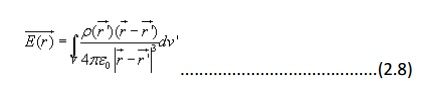Similar technique can be adopted when the charge distribution is in the form of a line charge density or a surface charge density.Electric flux density:

As stated earlier electric field intensity or simply ‘Electric field' gives the strength of the field at a particular point. The electric field depends on the

material media in which the field is being considered. The flux density vector is defined to be independent of the material media (as we'll see that it relates to the charge that is producing it).For a linear isotropic medium under consideration; the flux density vector is defined as: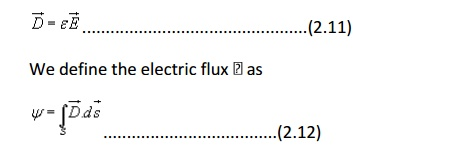Gauss's Law: Gauss's law is one of the fundamental laws of electromagnetism and it states that the total electric flux through a closed surface is equal to the total charge enclosed by the surface.Let us consider a point charge Q located in an isotropic homogeneous medium of dielectric constant . The flux density at a distance r on a surface enclosing the charge is given byIf we consider an elementary area ds, the amount of flux passing through the elementary area is given bywhich can seen to be same as what we have stated in the definition of Gauss's Law.

Application of Gauss's Law1.An infinite line charge

As the first example of illustration of use of Gauss's law, let consider the problem of determination of the electric field produced by an infinite line charge of density L2C/m. Let us consider a line charge positioned along the z-axis as shown in Fig. 2.4(a) (next slide). Since the line charge is assumed to be infinitely long, the electric field will be of the form as shown in Fig. 2.4(b) (next slide).

If we consider a close cylindrical surface as shown in Fig. 2.4(a), using Gauss's theorm we can write,Considering the fact that the unit normal vector to areas S1 and S3 are perpendicular to the electric field, the surface integrals for the top and bottom surfaces evaluates to zero. Hence we can write,2. Infinite Sheet of Charge

As a second example of application of Gauss's theorem, we consider an infinite charged sheet covering the x-z plane as shown in figure 2.5.

Assuming a surface charge density of rs for the infinite surface charge, if we consider a cylindrical volume having sides Ds placed symmetrically as shown in figure 5, we can write:It may be noted that the electric field strength is independent of distance. This is true for the infinite plane of charge; electric lines of force on either side of the charge will be perpendicular to the sheet and extend to infinity as parallel lines. As number of lines of force per unit area gives the strength of the field, the field becomes independent of distance. For a finite charge sheet, the field will be a function of distance.

3. Uniformly Charged SphereBy applying Gauss's theorem,Electrostatic Potential and Equipotential Surfaces

In the previous sections we have seen how the electric field intensity due to a charge or a charge distribution can be found using Coulomb's law or Gauss's law. Since a charge placed in the vicinity of another charge (or in other words in the field of other charge) experiences a force, the movement of the charge represents energy exchange. Electrostatic potential is related to the work done in carrying a charge from one point to the other in the presence of an electric field.

Let us suppose that we wish to move a positive test charge Dc2 from a point to another point Q as shown in the Fig. 2.8.

The force at any point along its path would cause the particle to accelerate and move it out of the region if unconstrained. Since we are dealing with an electrostatic case, a force equal to the negative of that acting on the charge is to be applied while  Dc2 moves from P to Q. The work done by this external agent in moving the charge by a distance Bar dl is given by:The negative sign accounts for the fact that work is done on the system by the external agent.The potential difference between two points P and Q , VPQ, is defined as the work done per unit charge, i.e.It may be noted that in moving a charge from the initial point to the final point if the potential difference is positive, there is a gain in potential energy in the movement, external agent performs the work against the field. If the sign of the potential difference is negative, work is done by the field.

We will see that the electrostatic system is conservative in that no net energy is exchanged if the test charge is moved about a closed path, i.e. returning to its initial position. Further, the potential difference between two points in an electrostatic field is a point function; it is independent of the path taken. The potential difference is measured in Joules/Coulomb which is referred to as Volts.

Let us consider a point charge Q as shown in the Fig. 2.9.Further consider the two points A and B as shown in the Fig. 2.9. Considering the movement of a unit positive test charge from B to A , we can write an expression for the potential difference as:It is customary to choose the potential to be zero at infinity. Thus potential at any point ( rA = r) due to a point charge Q can be written as the amount of work done in bringing a unit positive charge from infinity to that point (i.e. rB = 0).Let us now consider a situation where the point charge Q is not located at the origin as shown in Fig. 2.10.So far we have considered the potential due to point charges only. As any other type of charge distribution can be considered to be consisting of point charges, the same basic ideas now can be extended to other types of charge distribution also. Let us first consider N point charges Q1, Q2,.....QN located at points withdepending on whether the charge distribution is linear, surface or a volume charge distribution and the summation is replaced by an integral. With these modifications we can write:It may be noted here that the primed coordinates represent the source coordinates and the unprimed coordinates represent field point.

Further, in our discussion so far we have used the reference or zero potential at infinity. If any other point is chosen as reference, we can write: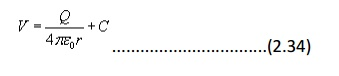where C is a constant. In the same manner when potential is computed from a known electric field we can write:The potential difference is however independent of the choice of reference.We have mentioned that electrostatic field is a conservative field; the work done in moving a charge from one point to the other is independent of the path. Let us consider moving a charge from point P1 to P2 in one path and then from point P2 back to P1 over a different path. If the work done on the two paths were different, a net positive or negative amount of work would have been done when the body returns to its original position P1. In a conservative field there is no mechanism for dissipating energy corresponding to any positive work neither any source is present from which energy could be absorbed in the case of negative work. Hence the question of different works in two paths is untenable, the work must have to be independent of path and depends on the initial and final positions.

Since the potential difference is independent of the paths taken, VAB = - VBA , and over a closed path,Example: Electric Dipole

An electric dipole consists of two point charges of equal magnitude but of opposite sign and separated by a small distance.

As shown in figure 2.11, the dipole is formed by the two point charges Q and -Q separated by a distance d , the charges being placed symmetrically about the origin. Let us consider a point P at a distance r, where we are interested to find the field.The potential at P due to the dipole can be written as:It may be noted that while potential of an isolated charge varies with distance as 1/r that of an electric dipole varies as 1/r2 with distance.The electric field for the dipole centered at the origin can be computed asis the magnitude of the dipole moment. Once again we note that the electric field of electric dipole varies as 1/r3 where as that of a point charge varies as 1/r2.

Equipotential Surfaces

An equipotential surface refers to a surface where the potential is constant. The intersection of an equipotential surface with an plane surface results into a path called an equipotential line. No work is done in moving a charge from one point to the other along an equipotential line or surface.

In figure 2.12, the dashes lines show the equipotential lines for a positive point charge. By symmetry, the equipotential surfaces are spherical surfaces and the equipotential lines are circles. The solid lines show the flux lines or electric lines of force.Michael Faraday as a way of visualizing electric fields introduced flux lines. It may be seen that the electric flux lines and the equipotential lines are normal to each other.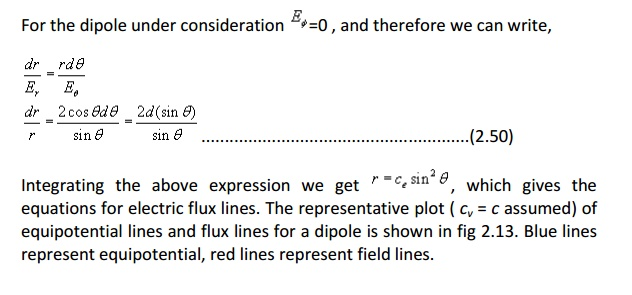Boundary conditions for Electrostatic fields

In our discussions so far we have considered the existence of electric field in the homogeneous medium. Practical electromagnetic problems often involve media with different physical properties. Determination of electric field for such problems requires the knowledge of the relations of field quantities at an interface between two media. The conditions that the fields must satisfy at the interface of two different media are referred to as boundary conditions .

In order to discuss the boundary conditions, we first consider the field behavior in some common material media.

In general, based on the electric properties, materials can be classified into three categories: conductors, semiconductors and insulators (dielectrics). In conductor , electrons in the outermost shells of the atoms are very loosely held and they migrate easily from one atom to the other. Most metals belong to this group. The electrons in the atoms of insulators or dielectrics remain confined to their orbits and under normal circumstances they are not liberated under the influence of an externally applied field. The electrical properties of semiconductors fall between those of conductors and insulators since semiconductors have very few numbers of free charges.

The parameter conductivity is used characterizes the macroscopic electrical property of a material medium. The notion of conductivity is more important in dealing with the current flow and hence the same will be considered in detail later on.

If some free charge is introduced inside a conductor, the charges will experience a force due to mutual repulsion and owing to the fact that they are free to move, the charges will appear on the surface. The charges will redistribute themselves in such a manner that the field within the conductor is zero. Therefore, under steady condition, inside a conductorThe surface charge distribution on a conductor depends on the shape of the conductor. The charges on the surface of the conductor will not be in equilibrium if there is a tangential component of the electric field is present, which would produce movement of the charges. Hence under static field conditions, tangential component of the electric field on the conductor surface is zero. The electric field on the surface of the conductor is normal everywhere to the surface . Since the tangential component of electric field is zero, the conductor surface is an equipotential surface. Asinside the conductor, the conductor as a whole has the same potential. We may further note that charges require a finite time to redistribute in a conductor. However, this time is very small ~10-15 sec for good conductor like copper.

Fig 2.14: Boundary Conditions for at the surface of a Conductor

Let us now consider an interface between a conductor and free space as shown in the figure 2.14.Let us consider the closed path pqrsp for which we can write,Therefore, we can summarize the boundary conditions at the surface of a conductor as: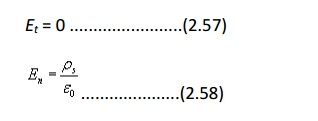Behavior of dielectrics in static electric field: Polarization of dielectric

Here we briefly describe the behavior of dielectrics or insulators when placed in static electric field. Ideal dielectrics do not contain free charges. As we know, all material media are composed of atoms where a positively charged nucleus (diameter ~ 10-15m) is surrounded by negatively charged electrons (electron cloud has radius ~ 10-10m) moving around the nucleus. Molecules of dielectrics are neutral macroscopically; an externally applied field causes small displacement of the charge particles creating small electric dipoles.These induced dipole moments modify electric fields both inside and outside dielectric material.

Molecules of some dielectric materials posses permanent dipole moments even in the absence of an external applied field. Usually such molecules consist of two or more dissimilar atoms and are called polar molecules. A common example of such molecule is water molecule H2O. In polar molecules the atoms do not arrange themselves to make the net dipole moment zero. However, in the absence of an external field, the molecules arrange themselves in a random manner so that net dipole moment over a volume becomes zero. Under the influence of an applied electric field, these dipoles tend to align themselves along the field as shown in figure 2.15. There are some materials that can exhibit net permanent dipole moment even in the absence of applied field. These materials are called electrets that made by heating certain waxes or plastics in the presence of electric field. The applied field aligns the polarized molecules when the material is in the heated state and they are frozen to their new position when after the temperature is brought down to its normal temperatures. Permanent polarization remains without an externally applied field.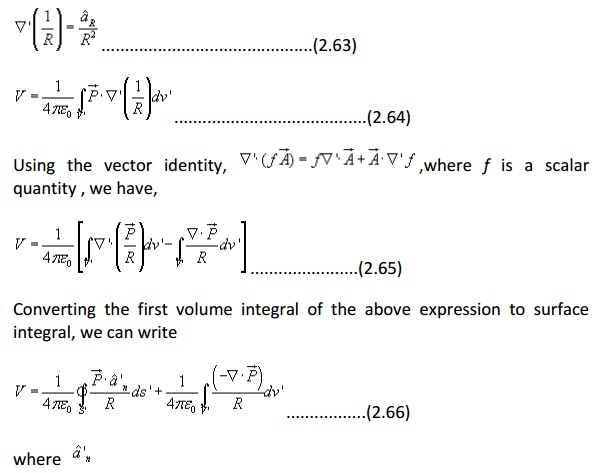is the outward normal from the surface element ds' of the dielectric. From the above expression we find that the electric potential of a polarized dielectric may be found from the contribution of volume and surface charge distributions having densitiesThese are referred to as polarisation or bound charge densities. Therefore we may replace a polarized dielectric by an equivalent polarization surface charge density and a polarization volume charge density. We recall that bound charges are those charges that are not free to move within the dielectric material, such charges are result of displacement that occurs on a molecular scale during polarization. The total bound charge on the surface is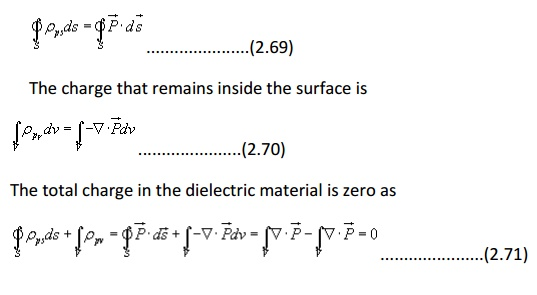When the dielectric properties of the medium are linear and isotropic, polarisation is directly proportional to the applied field strength andFor crystals, the reference coordinates can be chosen along the principal axes, which make off diagonal elements of the permittivity matrix zero. Therefore, we have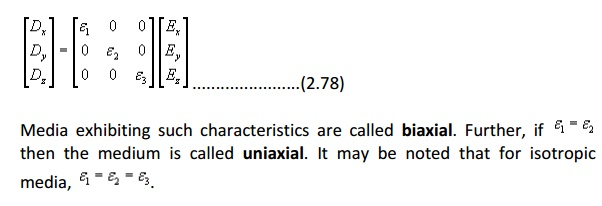Lossy dielectric materials are represented by a complex dielectric constant, the imaginary part of which provides the power loss in the medium and this is in general dependant on frequency.

Another phenomenon is of importance is dielectric breakdown. We observed that the applied electric field causes small displacement of bound charges in a dielectric material that results into polarization. Strong field can pull electrons completely out of the molecules. These electrons being accelerated under influence of electric field will collide with molecular lattice structure causing damage or distortion of material. For very strong fields, avalanche breakdown may also occur. The dielectric under such condition will become conducting.

The maximum electric field intensity a dielectric can withstand without breakdown is referred to as the dielectric strength of the material.

Boundary Conditions for Electrostatic Fields:

Let us consider the relationship among the field components that exist at the interface between two dielectrics as shown in the figure 2.17. The permittivity of the medium 1 and medium 2 arewhere Et and En are the tangential and normal components of the electric field respectively.

Let us assume that the closed path is very small so that over the elemental path length the variation of E can be neglected. Moreover very near to the interface,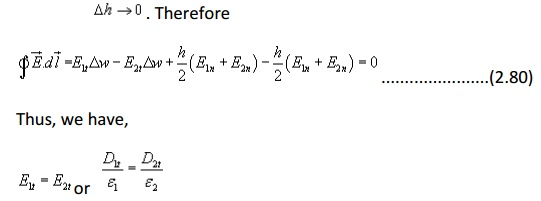i.e. the tangential component of an electric field is continuous across the interface.

For relating the flux density vectors on two sides of the interface we apply Gauss’s law to a small pillbox volume as shown in the figure. Once again as Dh->0, we can writeThus we find that the normal component of the flux density vector D is discontinuous across an interface by an amount of discontinuity equal to the surface charge density at the interface.

Example

Two further illustrate these points; let us consider an example, which involves the refraction of D or E at a charge free dielectric interface as shown in the figure 2.18.

Using the relationships we have just derived, we can writeFig 2.18: Refraction of D or E at a Charge Free Dielectric Interface

Capacitance and Capacitors

We have already stated that a conductor in an electrostatic field is an Equipotential body and any charge given to such conductor will distribute themselves in such a manner that electric field inside the conductor vanishes. If an additional amount of charge is supplied to an isolated conductor at a given potential, this additional charge will increase thewhere the constant of proportionality C is called the capacitance of the isolated conductor. SI unit of capacitance is Coulomb/ Volt also called Farad denoted by F. It can It can be seen that if V=1, C = Q. Thus capacity of an isolated conductor can also be defined as the amount of charge in Coulomb required to raise the potential of the conductor by 1 Volt.

Of considerable interest in practice is a capacitor that consists of two (or more) conductors carrying equal and opposite charges and separated by some dielectric media or free space. The conductors may have arbitrary shapes. A two-conductor capacitor is shown in figure 2.19.When a d-c voltage source is connected between the conductors, a charge transfer occurs which results into a positive charge on one conductor and negative charge on the other conductor. The conductors are equipotential surfaces and the field lines are perpendicular to the conductor surface. If V is the mean potential difference between the conductors, the capacitanceFor the parallel plate capacitor shown in the figure 2.20, let each plate has area A and a distance h separates the plates. A dielectric of permittivity e  fills the region between the plates. The electric field lines are confined between the plates. We ignore the flux fringing at the edges of the plates and charges are assumed to be uniformly distributed over the conductingSeries and parallel Connection of capacitors

Capacitors are connected in various manners in electrical circuits; series and parallel connections are the two basic ways of connecting capacitors. We compute the equivalent capacitance for such connections.

Series Case: Series connection of two capacitors is shown in the figure 2.21. For this case we can write,The same approach may be extended to more than two capacitors connected in series.

Parallel Case: For the parallel case, the voltages across the capacitors are the same.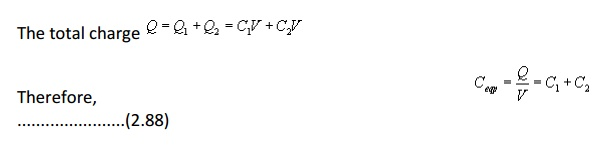Electrostatic Energy and Energy Density

We have stated that the electric potential at a point in an electric field is the amount of work required to bring a unit positive charge from infinity (reference of zero potential) to that point. To determine the energy that is present in an assembly of charges, let us first determine the amount of work required to assemble them. Let us consider a number of discrete charges Q1Q2,......., QN are brought from infinity to their present position one by one. Since initially there is no field present, the amount of work done in bring Q1 is zero. Q2 is brought in the presence of the field of Q1, the work done W1Q2V21 where V21 is the potential at the location of Q2 due to Q1. Proceeding in this manner, we can write, the total work doneIf instead of discrete charges, we now have a distribution of charges over a volume v then we can write,Poisson’s and Laplace’s Equations

For electrostatic field, we have seen that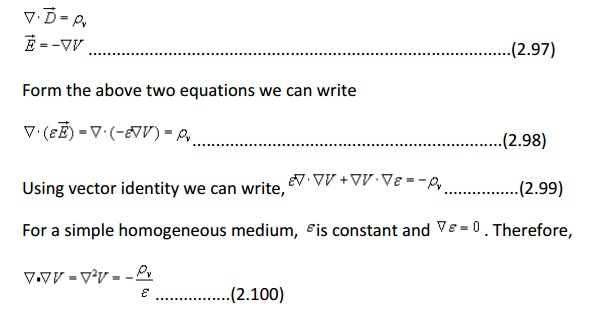Therefore, in Cartesian coordinates, Poisson equation can be written as:which is known as Laplace’s equation.

Laplace’s and Poisson’s equation are very useful for solving many practical electrostatic field problems where only the electrostatic conditions (potential and charge) at some boundaries are known and solution of electric field and potential is to be found throughout the volume. We shall consider such applications in the section where we deal with boundary value problems.

ASSIGNMENT PROBLEMS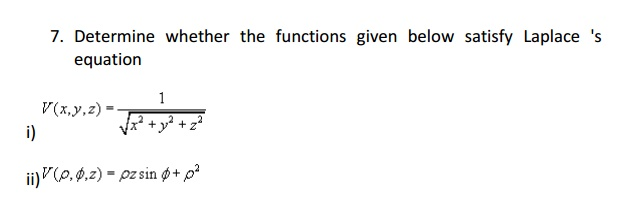Study Material, Lecturing Notes, Assignment, Reference, Wiki description explanation, brief detail
Electromagnetic Theory : Electrostatics |